# Introduction to exponential Smoothing for Time Series Forecasting using Python

Exponential Smoothing is a time series forecasting method for univariate data, that can be extended to support data which has trend & seasonal components.

Exponential smoothing forecasting methods are similar in that a prediction is a weighted sum of past observations, but the model explicitly uses an exponentially decreasing weight for past observations. Specifically, past observations are weighted with a geometrically decreasing ratio.

## Types of Exponential Smoothing¶

There are three main types of exponential smoothing time series forecasting methods.

### Simple Exponential Smoothing¶

Simple Exponential Smoothing, is a time series forecasting method for univariate data which does not consider the trend and seasonality in the input data while forecasting. The prediction is just the weighted sum of past observations.
It requires a single parameter, called alpha ($\alpha$), also called the smoothing factor.

This parameter controls the rate at which the influence of the observations at prior time steps decay exponentially. Alpha is often set to a value between 0 and 1. Large values mean that the model pays attention mainly to the most recent past observations, whereas smaller values mean more of the history is taken into account when making a prediction.

Hyper Parameter:

• $\alpha$ – Smoothing factor for the level.

Let’s see Python example for Simple exponential Smoothing.

For this example I am using the Airline passengers dataset prvided by Kaggle. You can download the dataset from here.

In :
import pandas as pd
import numpy as np
import matplotlib.pyplot as plt
%matplotlib inline

df.index.freq = 'MS'

Out:
#Passengers
Month
1949-01-01 112
1949-02-01 118
1949-03-01 132
1949-04-01 129
1949-05-01 121

## Train and Test data Splitting¶

In :
train_data = df.iloc[:108]
test_data = df.iloc[108:]


Lets plot the Train and Test data

In :
train_data['#Passengers'].plot(legend=True,label='TRAIN')
test_data['#Passengers'].plot(legend=True,label='TEST',figsize=(12,8));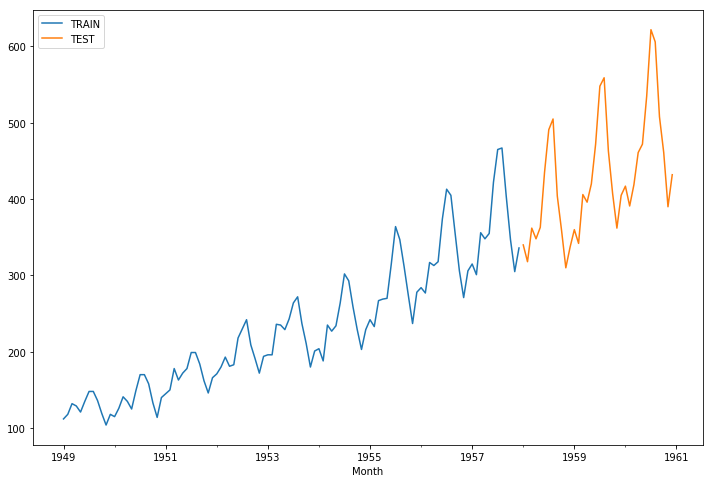From the above plot, it is clearly visible that the Airline passengers data has upward trend and seasonility(approx 12 Months). We can also get the Seasonality and Trend using seasonal_decompose module in Python

In :
from statsmodels.tsa.seasonal import seasonal_decompose
seasonal_decompose(train_data['#Passengers']).plot();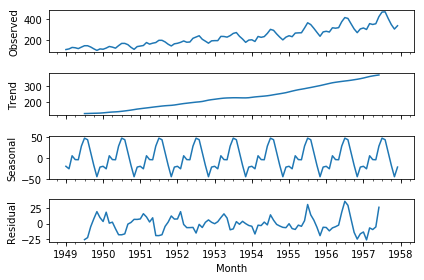I will implement simple smoothing in Python via the SimpleExpSmoothing Statsmodels class. For choosing the $\alpha$ value, please refer this link.

In :
from statsmodels.tsa.holtwinters import SimpleExpSmoothing
span = 12 # The model will consider the last 12 months weighted average for forecasting
alpha = 2/(span+1)
model = SimpleExpSmoothing(train_data['#Passengers']).fit(smoothing_level=alpha)
test_predictions = model.forecast(36).rename('SES Forecast')


Now lets plot the Predictions.

In :
train_data['#Passengers'].plot(legend=True,label='TRAIN')
test_data['#Passengers'].plot(legend=True,label='TEST',figsize=(12,8))
test_predictions.plot(legend=True,label='PREDICTION');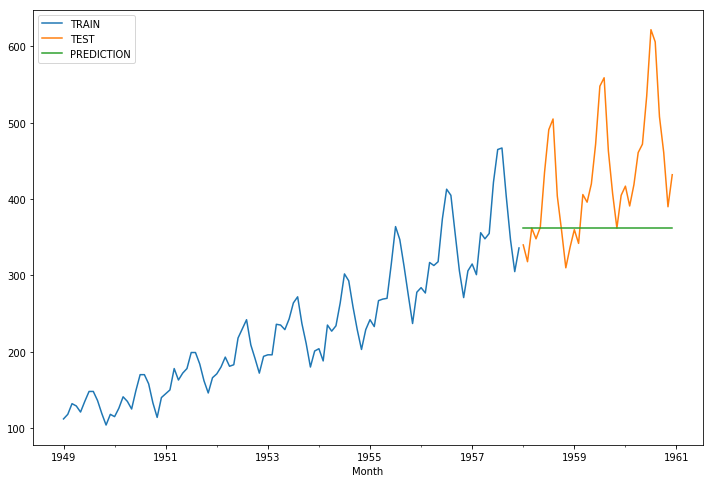In :
from sklearn.metrics import mean_squared_error
rms_error = np.sqrt(mean_squared_error(test_data['#Passengers'],test_predictions))
print("RMS Error is ",rms_error)

RMS Error is  102.76000445874031


As stated earlier, the SimpleExponentialModel does not consider the trend and seasonality. It will just take the weighted average of past data and forecast that average for all testing data. That’s why you can observe a straight line as the prediction. This model is not much useful for us.

### Double Exponential Smoothing¶

Double Exponential Smoothing is an extension to Simple Exponential Smoothing that explicitly adds support for trends in the univariate time series.

In addition to the alpha parameter for controlling smoothing factor for the level, an additional smoothing factor is added to control the decay of the influence of the change in trend called beta ($\beta$).

The method supports trends that change in different ways: an additive and a multiplicative, depending on whether the trend is linear or exponential respectively.

Double Exponential Smoothing with an additive trend is classically referred to as Holt’s linear trend model, named for the developer of the method Charles Holt.

Additive Trend: Double Exponential Smoothing with a linear trend.

Multiplicative Trend: Double Exponential Smoothing with an exponential trend.
For longer range (multi-step) forecasts, the trend may continue on unrealistically. As such, it can be useful to dampen the trend over time.

Hyperparameters:

• Alpha: Smoothing factor for the level.
• Beta: Smoothing factor for the trend.

I will implement Double Exponential Smoothing in Python via the ExponentialSmoothing Statsmodels class. Here I am not choosing the parameters $\alpha$ and $\beta$. The model will use default values.

In :
from statsmodels.tsa.holtwinters import ExponentialSmoothing
test_predictions = double_model.forecast(36).rename('DES Forecast')


Now, lets plot the predictions.

In :
train_data['#Passengers'].plot(legend=True,label='TRAIN')
test_data['#Passengers'].plot(legend=True,label='TEST',figsize=(12,8))
test_predictions.plot(legend=True,label='PREDICTION');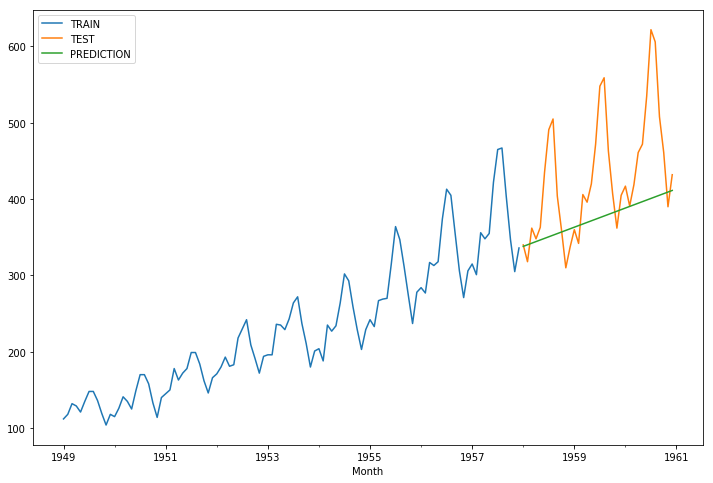In :
rms_error = np.sqrt(mean_squared_error(test_data['#Passengers'],test_predictions))
print("RMS Error for DES is ",rms_error)

RMS Error for DES is  87.72516049519773


As stated earlier, Double Exponential Smoothing will consider only the trend and ignore the seasonality. Since the data has upward trend, the prediction is a straight line with positive Slope.

### Triple Exponential Smoothing¶

Triple Exponential Smoothing is an extension of Double Exponential Smoothing that explicitly adds support for seasonality to the univariate time series.

This method is sometimes called Holt-Winters Exponential Smoothing, named for two contributors to the method: Charles Holt and Peter Winters.

In addition to the alpha and beta smoothing factors, a new parameter is added called gamma ($\gamma$) that controls the influence on the seasonal component.

As with the trend, the seasonality may be modeled as either an additive or multiplicative process for a linear or exponential change in the seasonality.

Additive Seasonality: Triple Exponential Smoothing with a linear seasonality.

Multiplicative Seasonality: Triple Exponential Smoothing with an exponential seasonality

Triple exponential smoothing is the most advanced variation of exponential smoothing and through configuration, it can also develop double and single exponential smoothing models.

Additionally, to ensure that the seasonality is modeled correctly, the number of time steps in a seasonal period (Period) must be specified. For example, if the series was monthly data and the seasonal period repeated each year, then the Period=12.

Hyperparameters:

• Alpha: Smoothing factor for the level.
• Beta: Smoothing factor for the trend.
• Gamma: Smoothing factor for the seasonality.
• Trend Type: Additive or multiplicative.
• Seasonality Type: Additive or multiplicative.
• Period: Time steps in seasonal period.

I will implement Triple Exponential Smoothing in Python via the ExponentialSmoothing Statsmodels class. Here I am not choosing the parameters $\alpha$, $\beta$ and $\gamma$. The model will use default values.

In :
from statsmodels.tsa.holtwinters import ExponentialSmoothing
test_predictions = triple_model.forecast(36).rename('TES Forecast')


Now, lets plot the predictions.

In :
train_data['#Passengers'].plot(legend=True,label='TRAIN')
test_data['#Passengers'].plot(legend=True,label='TEST',figsize=(12,8))
test_predictions.plot(legend=True,label='PREDICTION');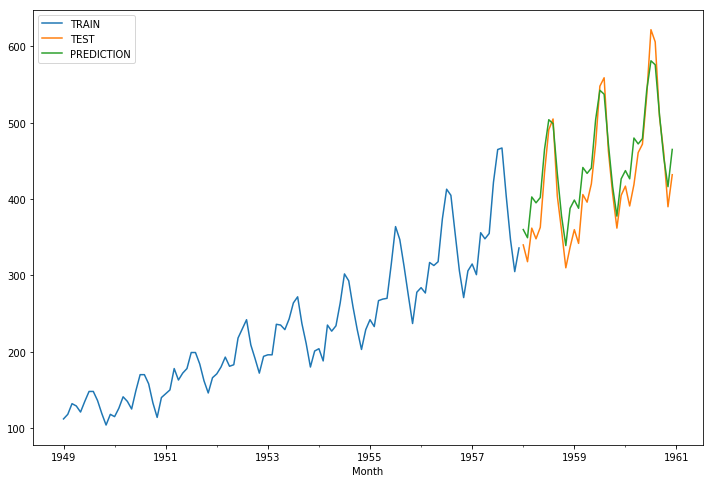In :
rms_error = np.sqrt(mean_squared_error(test_data['#Passengers'],test_predictions))
print("RMS Error for TES is ",rms_error)

RMS Error for TES is  29.70690700235816


Since Triple Exponential Model is considering the trend and Seasonality, it is able to give very close forecasts. Even the RMS error also very less and improved a lot compared to SES & DES.